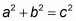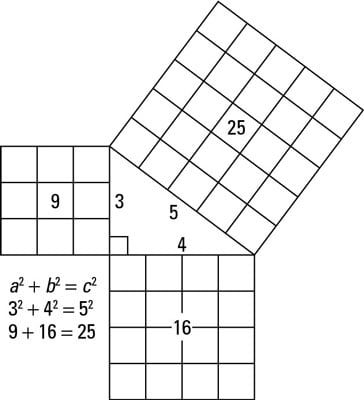##### Geometry For DummiesThe Pythagorean Theorem has been known for at least 2,500 years. You use the Pythagorean Theorem when you know the lengths of two sides of a right triangle and you want to figure out the length of the third side.

The Pythagorean Theorem: The sum of the squares of the legs of a right triangle is equal to the square of the hypotenuse:Here, a and b are the lengths of the legs and c is the length of the hypotenuse. The legs are the two short sides that touch the right angle, and the hypotenuse (the longest side) is opposite the right angle.The preceding figure shows how the Pythagorean Theorem works for a right triangle with legs of 3 and 4 and a hypotenuse of 5.